### Handling of Missing Data

Subsections:

PROC MCMC automatically augments missing values via the use of the MODEL statement. PROC MCMC treats missing values as unknown parameters, assigns distributions to the variables, and incorporates the sampling of the missing data as part of Markov chain.

(In SAS/STAT 9.3 and earlier releases, by default, PROC MCMC discarded all observations that had missing or partial missing values. PROC MCMC could not model missing values.)

You can use the MISSING= option in the PROC MCMC statement to specify how you want PROC MCMC to handle the missing values. If you specify MISSING=CC (CC stands for complete cases), PROC MCMC discards all observations that have missing or partial missing values before carrying out the simulation. If you specify MISSING=AC (AC stands for all cases), PROC MCMC neither discards any missing values nor augments them.

Generally speaking, there are three types of missing data models, as discussed by Rubin (1976). Also see Little and Rubin (2002) for a comprehensive treatment of missing data analysis. The rest of this section provides an overview of these three types of missing data models and explains how to use PROC MCMC to fit them.

#### Missing Completely at Random (MCAR)

Data are said to be MCAR if the probability of a missing value (or the failure of observing a value) does not depend on any other observations in the data set, regardless of whether they are observed or missing. That is, the observed and unobserved values are independent of each other: ifis missing, it is MCAR if the probability of observingis independent of other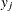(and other covariates) in the data set. Under this assumption, both the observed and unobserved data are random samples of all the data; hence, fitting a model based only on the observed data does not introduce any biases. This type of analysis is called a complete-case analysis. To carry out a complete-case analysis, you must specify MISSING=CC in the PROC MCMC statement. (In SAS/STAT 9.3 and earlier, PROC MCMC performed a complete-case analysis when the data contained missing values.)

#### Missing at Random (MAR)

Data are said to be MAR if the probability of a missing value can depend on some observed quantities but does not depend on any unobserved data. For example, suppose thatare completely observed for all observations and someare missing. MAR states that the probability of observingis independent of other missing(values that could have been observed) and that it depends only on(and, potentially, observed).

The MAR assumption states that the missingare no longer random samples and that they need to be modeled (via the likelihood specification of the missing values). At the same time, the independence assumption of the missing values on the unobserved quantities states that the missing mechanism (usually an binary indicator variable such that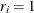ifis missing and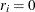otherwise) can be ignored and does not need to be taken into account. Hence, MAR is sometimes referred to as ignorably missing. It is not the missing values that can be ignored, it is the missing mechanism that can be ignored.

By default, PROC MCMC treats the missing data as MAR (this assumes that you do not input a binary indicator variable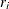and model it specifically): each missing value becomes an extra parameter and PROC MCMC updates it in every iteration. PROC MCMC assumes that both the missing values and observed values arise from the same distribution (which is specified in the MODEL statement),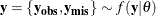where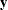consists of observed (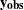) and missing (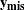) values, and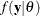is the likelihood function with parameters.

You can use the MODEL statement to model missing covariates. Using multiple MODEL statements enables you to specify, for example, a marginal distribution for missing values in covariate x and a conditional distribution for the response variable y given x as follows:

model x ~ normal(alpha, var=s2_x);
model y ~ normal(beta * x, var=s2_y);


In each iteration, PROC MCMC draws samples for every missing value in variable x, then every missing value in variable y, conditional on the drawn values of the x variable.

#### Missing Not at Random (MNAR)

Data are said to be MNAR if the probability of a missing value depends on unobserved data (or data that could have been observed): the probability thatis missing depends on the missing values of other. This is a very general scenario that assumes that the missing mechanism is no longer ignorable (it is sometimes referred to as nonignorably missing) and that a model for the missing mechanism is required in order to make correct inferences about the model parameters.

Let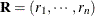be the missing value indicator for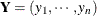, whereifis missing andotherwise. Thisis usually part of an input data set where you preprocess the response variable and create this missing value indicator variable. Modeling MNAR data implies that you must specify a joint likelihood function over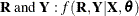, whererepresents the covariates andrepresents the model parameters. This joint distribution can be factored in two ways: a pattern-mixture model and a selection model.

The selection model factors the joint distributionand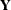into a marginal distribution forand a conditional distribution for,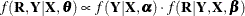where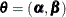,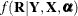is usually a binary model with a logit or probit link that involves regression parameters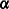, and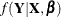is the sampling distribution that generateswith model parameters.

The pattern-mixture model factors the opposite way, a marginal distribution forand a conditional distribution for,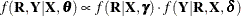where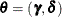.

You can use PROC MCMC to fit either model by specifying multiple MODEL statements: one for the marginal distribution and one for the conditional distribution. Suppose that the variable r is the missing data indicator, which is modeled using a logit model, and that the response variable y is a Poisson regression that includes the missing variable indicator as one of its covariates. The following statements are a PROC MCMC program that fits a pattern-mixture model:

pi = logistic(alpha * x1);
model r ~ binary(pi);
mu = beta0 + beta1 * x2 + beta3 * r;
model y ~ poisson(exp(mu));


The first MODEL statement uses a binary model with logit link to model the missing mechanism, and the second MODEL statement models the response variable with a Poisson regression that includes the missing value indicator as one of its covariates. Each of the two sets of regression has its covariates and regression coefficients. If this hypothetical data set contained missing values in covariates x1 and x2, you could add two more MODEL statements to handle each variable as follows:

model x1 ~ normal(mu1, var=s2_x1);
pi = logistic(alpha * x1);
model r ~ binary(pi);
model x2 ~ normal(mu2, var=s2_x2);
mu = beta0 + beta1 * x2 + beta3 * r;
model y ~ poisson(exp(mu));


 A missing value is usually, although not necessarily, represented by a single period (.) in the input data set.